# The Exterior Angle Theorem - an appreciation

Scott E. Brodie
August 14, 2000

The Exterior Angle Theorem (Euclid I.16), "In any triangle, if one of the sides is produced, then the exterior angle is greater than either of the interior and opposite angles," is one of the cornerstones of elementary geometry. In many contemporary high-school texts, the Exterior Angle Theorem appears as a corollary of the famous result (equivalent to the Parallel Postulate) that the three angles of a triangle sum to two right angles -- (since adjacent interior and exterior angles are supplementary, the sum of the two remote interior angles equals the exterior angle, which must thus be greater than either one alone).

In contrast, in Euclid's sequence of propositions, the Exterior Angle Theorem appears before any invocation of the Parallel Postulate. [It is a great credit to Euclid's sense of parsimony that by delaying any use of the Parallel Postulate as long as possible, he helps draw the distinction between those parts of geometry which are valid independent of the Parallel Postulate (so-called "absolute" or "neutral" geometry, and those which depend on it. Euclid thus almost eerily anticipates the development of "Non-Euclidean Geometry" over two thousand years after his own time.] Thus the Exterior Angle Theorem, and many of its consequences, apply more generally than one might suppose from the watered-down "High School" treatment.

The importance of the Exterior Angle Theorem can be seen by listing some of the important theorems which derive directly from it:

• In any triangle, the sum of any two angles is less than two right angles (Euclid I.17)
This is the beginning of the argument which leads to Legendre's Lemma: The sum of the three angles of a triangle are less than or equal to two right angles.

One can also use this proposition to simplify Euclid's enunciation of the Parallel Postulate: it becomes unnecessary to state that two lines cut by a transversal  "meet on that side on which are the angles less than the two right angles." If they met on the other side, they would form a triangle whose angle sum exceeds two right angles.

• No triangle can contain two right angles (or equivalently, the perpendicular to a given line through any external point is unique).
• "SAA" -  triangles are congruent in which two pairs of angles and a side not between them are, respectively, congruent.
From SAA follows the "Hypotenuse-Leg Theorem" that two right triangles are congruent in which hypotenuse and one leg in one triangle are, respectively, congruent to those of the other.
• A line cannot intersect a circle in three distinct points.
• All of the geometric inequalities in Euclid derive from the Exterior Angle Theorem:
In any triangle the angle opposite the greater side is greater. (Euclid I.18) (and conversely, Euclid I.19)
In any triangle the sum of any two sides is greater than the remaining one. (Euclid 1.20)
If two triangles have two sides equal to two sides respectively, but have one of the angles contained by the equal straight lines  greater than the other, then they also have the base greater than the base. (Euclid I.24) (and conversely, Euclid I.25)
• If a straight line falling on two straight lines makes the alternate angles equal to one another, then the straight lines are parallel  to one another. ("AIP", Euclid I.27)

•     It is therefore distressing to discover that Euclid's proof of the Exterior Angle Theorem is deeply flawed! It can charitably be described as a glib example of "reasoning from the diagram."

To see what can go wrong, let us recall Euclid's argument, and see what happens if we try to apply it to triangles drawn on the surface of a sphere.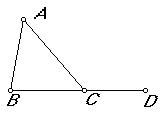Euclid shows that angle ACD is greater than angle A. He first bisects segment AC at E

This works perfectly well on the surface of a sphere.
He draws the ray from B through E to F, so that BE = EF, and connects F to C.
This works perfectly well on the surface of a sphere.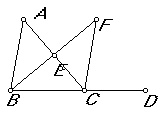He now argues that triangles AEB and CEF are congruent (SAS), since AE = EC, BE = EF, and angle AEB and CEF are equal, as they are vertical angles.

This works pretty well on the surface of a sphere. Vertical angles are equal on a sphere. SAS holds on a sphere so long as we agree to restrict the notion of "triangle"  to include only segments which are less than half the circumference of the sphere in length.
Euclid then simply proceeds, "But the angle ECD is greater than the angle ECF, therefore the angle ACD is greater than the angle BAE," with reference to Common Notion 5, "The whole is greater than the part." Evidently, he means to argue that, since the point F lies in the interior of angle ECD, the angle ECF is less than angle ECD.

This is where the proof would get into trouble on the surface of a sphere. Indeed, the observation that F lies in the interior of angle ECD is tantamount to assuming what it is we are trying to prove. On the sphere, the point F may come to lie on line CD, or even beyond it! (For example, consider the spherical triangle with three right angles.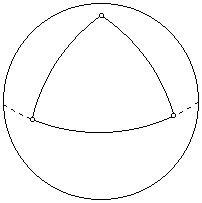For this triangle, AB, BC, and CA are all equal to 1/4 of the circumference of the sphere  in length. BE is also 1/4 of the circumference of the sphere, and is perpendicular to AC at E. Thus EF is also 1/4 of the circumference of the sphere, and BF is 1/2 the circumference of the sphere, and lies on the line CD. It may help to visualize this on a Globe: take A the intersection of the Prime Meridian with the Equator (a point in the South Atlantic near Ghana),  B as the North Pole,  and C as the point on the Equator at 90 degrees East Longitude, in the Indian Ocean near Sumatra. Then E will lie on the Equator at 45 degrees East Longitude, just offshore near Somalia, and F, at the South Pole, will lie on line CD, not in the interior of angle ACD.)

Indeed, on the sphere, the Exterior Angle Theorem and most of its consequences break down utterly. The exterior angles of the spherical triangle with three right angles are themselves right angles; this triangle contains three, let alone two, right angles; its angle sum exceeds two right angles. Similarly, AIP fails on the sphere: a walk along the Equator, making a left turn and following, say, the Prime Meridian up to the North Pole, followed by a right turn and a walk south along the meridian at 90 degrees east longitude, will bring you back to the Equator.

Apparently, Euclid's argument proves "too much". In order to clarify where matters stand on the plane, we will need to look more closely at how our axioms for plane geometry rule out the behavior we have seen on the sphere.

Perhaps the simplest argument is that adopted by [Hilbert, Theorem I.22]: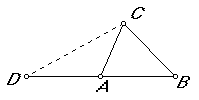Extend BA to D, so that AD = CB. Connect C to D. Suppose that angles CAD and ACB were equal. Then we would have triangle CAD congruent to triangle ACB (by SAS); in particular angle ACD would be congruent to angle CAB. But then, since angles DAC, CAB are supplementary, so too would angles ACD and ACB be supplementary, and points D, C, B would be collinear.

On the plane, this is a contradiction: the line through points D and B is unique, whereas  lines DAB and DCB must be distinct in order for ABC to be a triangle. On the other hand, on the sphere, many distinct lines (great circles) share the same two (antipodal) points, and the configuration entails no contradiction.

Thus, on the plane, we conclude that angles CAD and ACB are unequal. If angle DAC were less than angle ACB, it would be possible to construct a segment CE, in the interior of angle ACB, with E between A and B, such that angle DAC = angle ACE.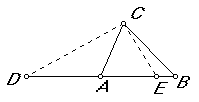Then, as before, we would have D,C,E collinear, resulting in two distinct lines connecting D to E, again a contradiction in the plane.

There remains only the possibility that angle DAC is greater than angle ACB, QED (in the plane).

(It is also possible to salvage Euclid's proof by means of careful use of a "plane separation" axiom, such as "In a plane, the set of points not on a line is the union of two disjoint sets such that (1) each set is convex and (2) if P is in one of these sets and Q is in the other, then the segment PQ intersects the line, see [Moise, Theorem 4.7]. Although an analogous separation axiom is valid on the sphere the modification of Euclid's proof breaks down on the spherical surface. AB: I am grateful to Professor Robert Foote of Wabash College for pointing out an ambiguity in the original wording of this remark.)

### References

1. D. W. Hendersen, Experiencing Geometry, Prentice-Hall, 2004
2. D. Hilbert, Foundations of Geometry, 2nd edition, La Salle, IL, Open Court, 1971
3. E. E. Moise, Elementary Geometry from an Advanced Standpoint, 3rd Ed. Reading, MA, Addison -Wesley 1990.